Technical Information Site of Power Supply Design

2019.01.10 DC/DC

# Example of Power Loss Calculation for a Power Supply IC

Examination of Losses

Up to this point we have calculated losses of areas where losses occur. In this article, we summarize these calculations and present an example of calculations of losses for a power supply IC.

Example of Power Loss Calculation for a Power Supply IC(Case of a synchronous rectification IC with an internal MOSFET)

The graphic below illustrates the relevant details from the standpoint of power supply IC losses. Here, the example is an IC with an internal MOSFET in the output stage. The blue portions are relevant here. The inductor is external, and so is omitted. When calculating the losses of the controller-type IC used in previous explanations, the losses of the MOSFET and the inductor are not included.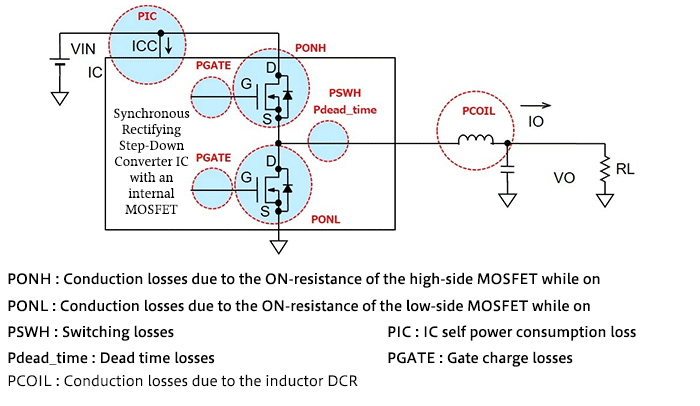In order to calculate losses, values in the terms of the equations used in calculations described above are needed. These are, in essence, the values appearing in data sheets.

In general, minimum, typical, and maximum values are given for data sheet specifications, that is, IC parameter values. Depending on the parameter, not all of the minimum, maximum and typical values are given; for example, only minimum or maximum value, or only typical value.

Which value to use requires consideration of various matters, but considering the scattering among values, losses should probably be calculated assuming worst-case conditions.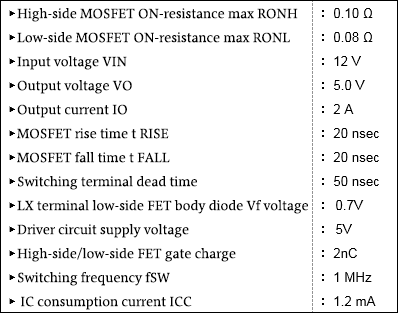Here, the values shown on the right are used. These values assume worst-case conditions. As the calculation procedure, the individual losses are calculated using the equations shown, and the losses are added.

① High-side MOSFET conduction loss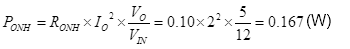② Low-side MOSFET conduction loss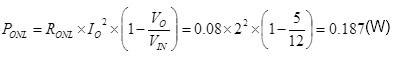③ High-side MOSFET switching loss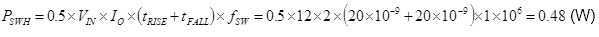④ Dead time loss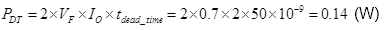⑤ Power consumption caused by IC control circuit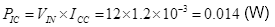⑥ Gate charge loss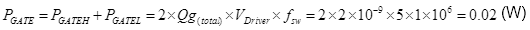Total of power losses for the power supply IC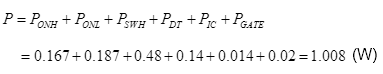In this example, power losses for the power supply IC are approximately 1 W. If the information necessary for calculations can be assembled, the calculations themselves are not particularly difficult.

#### Key Points:

・Power losses for a power supply IC are the total of the losses in various places.

・There are various approaches where the values used in calculations are concerned, but losses under worst-case conditions should be used in calculations.

This website uses cookies.

By continuing to browse this website without changing your web-browser cookie settings, you are agreeing to our use of cookies.